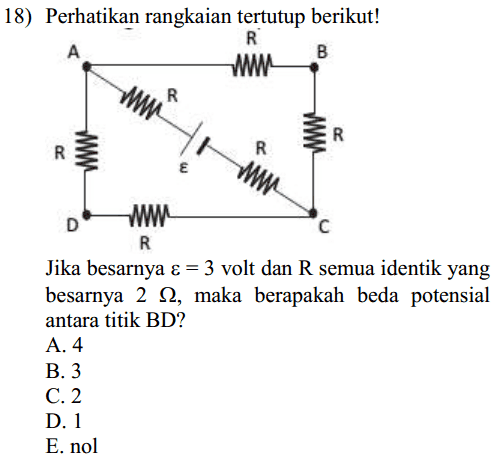# Potential difference between two points on a circuit

## Homework StatementThe circuit is shown as above.
If ε = 3 volt and each resistor has 2 ohm resistance, then what's the potential difference between point B and point D ?

A. 4
B. 3
C. 2
D. 1
E. 0

## Homework Equations

V = I R
R series = R1 + R2 + ..
1/ R parallel = 1/R1 + 1/R2 + ...

## The Attempt at a Solution

[/B]
The circuit is very weird. I don't even know how to start.

cnh1995
Homework Helper
Gold Member
Are you familiar with Kirchhoff's laws?

Are you familiar with Kirchhoff's laws?

Yes, of course.. KVL and KCL..
Okay, I'll use KVL for the two triangles

4IR = ε
I = ε/4R = 3/8 ampere

The current in triangle ABC also 3/8 ampere, right ? (The resistance are equal also the emf)

But, what's the potential difference between point B and D?

cnh1995
Homework Helper
Gold Member
Assume current through diagonal AC as i and current through ABC as i1. So, current through ADC is i-i1. Using these currents, write KVL and you'll get values for i and i1.
Actually, this problem can be solved by observation only, using the symmetry of the circuit.

•Samy_A
Assume current through diagonal AC as i and current through ABC as i1. So, current through ADC is i-i1. Using these currents, write KVL and you'll get values for i and i1.

##Triangle\ ABC \\
2I_1R+2IR - \varepsilon = 0 \\
4I_1 + 4I = 3\\
2((I-I_1)R) + 2IR - \varepsilon = 0 \\
4IR-2I_1R = 3 \\
8I - 4I_1 = 3 \\##

Solving the equation, I get i = 0.5 A, and i1 = 0.25 A, and it means that i2 = 0.25 A
How to determine the potential difference between B and D ??

Actually, this problem can be solved by observation only, using yhe symmetry of the circuit.

Yeah, I also see that it's kinda like the Wheatstone bridge, isn't it ??
But, eventually, the current through diagonal AC is not zero (not as I thought it would be in Wheatstone bridge)..

cnh1995
Homework Helper
Gold Member
Solving the equation, I get i = 0.5 A, and i1 = 0.25 A, and it means that i2 = 0.25 A
Good!
How to determine the potential difference between B and D ??
Let's say point A is at a potential Va and point B is at potential Vb. Voltage drop across the resistor between A and B is Va-Vb. What is the value of this voltage drop? You know the currents now.

Last edited:
Good!

Let's say point A is at a potential Va. You know the currents now. What is the potential of point B in terms of Va and known current and resistor values? (Hint:Ohm's law).

Vb = 3 - 0.5 * 2 - 0.25 * 2 = 1.5 V
Vd = 3 - 0.5 * 2 - 0.25 *2 = 1.5 V
The potential difference is zero, right ?

Anyway, is this circuit a Wheatstone bridge?

cnh1995
Homework Helper
Gold Member
The potential difference is zero, right ?
Right.
Anyway, is this circuit a Wheatstone bridge?
Yes. Supply is connected between A and C and output is taken between B and D.

•terryds